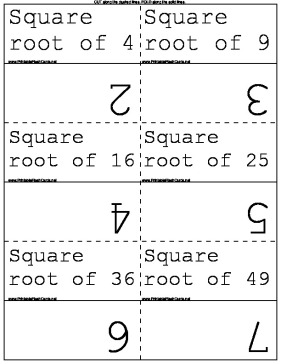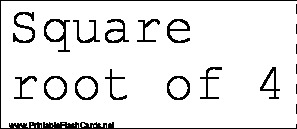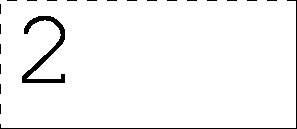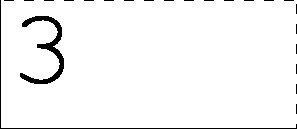Sample flash cards in this set:

Front:Back:This set includes the following cards:
Square root of 42
Square root of 93
Square root of 164
Square root of 255
Square root of 366
Square root of 497
Square root of 648
Square root of 819
Square root of 10010
Square root of 12111
Square root of 14412
Square root of 16913